# Frontiers in Space Technologies## ORIGINAL RESEARCH article

Front. Space Technol., 27 September 2021 | https://doi.org/10.3389/frspt.2021.696464

# A Hybrid Beamforming Design for Massive MIMO LEO Satellite Communications

• 1Electrical and Computing Engineering Department, North Carolina State University, Raleigh, NC, United States
• 2atlanTTic Research Center, Universidade de Vigo, Vigo, Spain
• 3Toyota Motor North America, Mountain View, CA, United States

5G and future cellular networks intend to incorporate low earth orbit (LEO) satellite communication systems (SatCom) to solve the coverage and availability problems that cannot be addressed by satellite-based or ground-based infrastructure alone. This integration of terrestrial and non terrestrial networks poses many technical challenges which need to be identified and addressed. To this aim, we design and simulate the downlink of a LEO SatCom compatible with 5G NR, with a special focus on the design of the beamforming codebook at the satellite side. The performance of this approach is evaluated for the link between a LEO satellite and a mobile terminal in the Ku band, assuming a realistic channel model and commercial antenna array designs, both at the satellite and the terminal. Simulation results provide insights on open research challenges related to analog codebook design and hybrid beamforming strategies, requirements of the antenna terminals to provide a given SNR, or required beam reconfiguration capabilities among others.

## 1 Introduction

Integrated satellite-terrestrial cellular networks are currently being pursued for long awaited commercial applications. The main expected benefit is an uninterrupted coverage, even in unserved/underserved areas. LEO constellations seem to be the most promising platforms for satellite-based non-terrestrial networks (NTNs), due to their relatively shorter propagation delay (Kodheli et al., 2017). Although this propagation delay between ground terminals and LEO satellites (3 ms at 1,000 km above the Earth surface) is non-negligible, LEO constellations can support continuity and ubiquity of the radio services, and also latency critical applications with requirements within tens of ms.

Beam configuration and reconfiguration in a link between a LEO satellite and a ground mobile terminal is challenging due to the long round trip delay, fast movement of the satellite and limited on board processing capabilities. Current LEO constellations (del Portillo et al., 2019) make use of fixed analog beams that illuminate a given area of the Earth’s surface, without any capability to steer narrow beams in the user directions. Digital precoding stages are designed independently of the analog beam with the purpose of reducing inter-beam interference among the beams illuminating adjacent regions of interest (ROI) (Devillers and Pérez-Neira, 2011). Moreover, these solutions assume that a single satellite is illuminating a large specific ROI, and handover procedures are put in place to enable satellite switching at the user side before the satellite movement causes loss of coverage.

Designing the LEO satellite footprint and the specific beam codebook at the satellite side in an integrated satellite-terrestrial network is challenging. The potential beampatterns need to be designed to guarantee coverage of the Earth’s surface, limit the inter-beam interference, maximize system throughput, and, at the same time, exhibit some degree of compatibility with conventional codebooks defined in cellular standards. Commercial efforts on deploying LEO constellations, not necessarily for integration with the terrestrial network, are described in (del Portillo et al., 2019; Xia et al., 2019). Sizes of the beam footprints for the different deployments are provided, but the details of the beam codebook are not available. The massive MIMO LEO satellite communication system designed in (You et al., 2020) considers the set of all feasible beams generated by a uniform planar array at the satellite rather than a codebook, without trying to reduce the inter-beam interference or the amount of on-board processing.

In this paper, we propose and evaluate a massive MIMO LEO satellite communication system operating in the Ku band, based on a hybrid beamforming architecture. In particular, we design first the footprint associated to a given LEO satellite. Then, we design the beam codebook for the hybrid beamforming stage such that the derived footprint is covered by the beams in the codebook. We build our design using a 2-D DFT-based grid of beams as in Type I and Type II 5G New Radio CSI codebooks (Miao et al., 2018; Ericsson, 2017a; Ericsson, 2017b; Samsung, 2017; Technical Specification Group Radio Access Network, 2017a; b), considering an oversampling factor to shape the available beampatterns to the size of the satellite footprint. Assuming this codebook at the satellite and commercially available SatCom antennas for the mobile terminals, we evaluate the coverage and throughput of this LEO massive MIMO system operating in the Ku band. Additional performance metrics such as SNR degradation over time or inter-beam interference are also evaluated. Numerical results provide the baseline performance of a state-of-the art massive MIMO LEO satellite communication system and allow the identification of open research challenges.

## 2 System Model

We consider the downlink of a massive MIMO LEO satellite communication system operating in the Ku band, as in current commercial deployments (del Portillo et al., 2019), and also compliant with the 3GPP proposal for 5G broadband satellite services (Angeletti and De Gaudenzi, 2020). We denote as $BDL$ the bandwidth allocated to the downlink. The system model we propose is general, but in the performance evaluation section we will focus on the Communications on the move (COOM) 5G use case. A given LEO satellite in the constellation can cover an elliptical region of interest (ROI), with semiradius $Rx$ and $Ry$, using a set of potential beams, as illustrated in Figure 1. Thus, the system supports the direct transmission to a maximum number of simultaneous mobile user terminals (UTs) on the ground, denoted as $Nu$, by using $Nb$ analog beams, with $Nu. Time or frequency division multiplexing mechanisms will enable beam sharing when more than one user per beam is simultaneously served. The beam footprints are not necessarily identical, to account for the different elevation angles across beams. We assume that the beams generated at the LEO satellite belong to a fixed codebook, i.e. the beams move with the satellite, as considered in Scenario C1 and D2 in the 3GPP technical report that specifies solutions for 5G NR to support NTNs (Technical Specification Group Radio Access Network, 2019). We also assume that the UTs know the position and trajectory of the satellites in the constellation at all times, as proposed in (Kim et al., 2020). This information is periodically updated and broadcasted by the LEO satellite operations center to the cellular network. Knowledge of the planned trajectory allows the UTs to obtain an estimate of the satellite position even at locations where the terrestrial network is not available. It is out of the scope of this paper to consider the impact of the position or trajectory errors in the system model.

FIGURE 1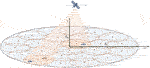FIGURE 1. System model for a LEO satellite communication system covering an elliptical area using a predefined beam codebook.

### 2.1 MIMO Architecture and Antenna Models

We assume that the satellite is equipped with a large uniform planar array (UPA) with dimension $Nxsat×Nysat$ and $Nxsat×Nysat$ denoting the total number of antennas. We consider $x$ to be the axis in the direction of the satellite’s movement and $y$ to be the axis in the direction orthogonal to the movement. The possible beamforming architectures for the LEO satellite include: fully digital, reconfigurable analog, analog based on beam switching and hybrid (Angeletti and De Gaudenzi, 2020). Our choice is a partially connected hybrid MIMO (Multi-Input Multi-Output) architecture with a fixed codebook-based analog stage, as a practical trade-off between complexity and reconfigurability. In this regard, a fully analogue reconfigurable beamforming network entails a large mass and power consumption, whereas a fully digital solution is costly and power hungry due to all the required connections. The advantages of the hybrid approach become especially relevant for very large arrays, with major efforts currently underway to develop massive hybrid arrays based on multiple subarrays operating with analog beam-forming (De Gaudenzi et al., 2020). The number of beamforming ports in each dimension is defined as $NxRF$ and $NyRF$ making a total of $NRF=NxRFNyRF$ RF-chains. The large array is organized into sub-arrays of size $Nxsub×Nysub$, with $Nxsub=Nxsat/NxRF,Nysub=Nysat/NyRF$ as illustrated in Figure 2. Each radio frequency (RF) chain drives one of the subarrays, generating an independent spot beam so the whole coverage area is illuminated. This approach allows the reduction of the number of beamforming ports and on board processing requirements, since the analog beams do not have to be constantly reconfigured.

FIGURE 2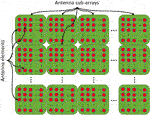FIGURE 2. Partially connected sub-array structure at the satellite antenna. Each subarray is controlled by a single RF-chain.

Assuming a hybrid architecture, the precoding matrix consisting on $Nb$ beam-patterns $F∈ℂNsat×Nb$ can be written as $F=FRFFBB$, where $FBB∈ℂNRF×Nb$ is the digital precoder and $FRF∈ℂNsat×NRF$ is the analog precoder. Due to power constraints in the mth RF-chain, we have that $||[FBB]m,:||2≤PRF$. We define the index function $IRF:{1,…,Nsat}→{1,…,NRX}$, where $IRF(n)$ is the index of the RF-chain to which the nth antenna is connected. With this definition, the RF precoder $FRF$ can be written as . We envision a digital precoder at the satellite to manage inter-beam interference in the context of a full frequency reuse system. Finally, it is also assumed that all the power amplifiers at the satellite UPA are identical, and their impact can be modeled as a scaling factor $P$ (Angeletti and De Gaudenzi, 2020).

For the antenna arrays at the mobile terminal, we consider three possible solutions that enable beamforming in the satellite direction. The first one is a uniform planar array (UPA) of moderate size, with dimensions $NxUT×NyUT$, having a total of $NUT=NxUTNyUT$ antenna elements. Recent advances on antenna technology enable the commercial applications of these planar arrays for SatCom terminals (Sikri and Jayasuriya, 2019). The second considered solution is a flat panel antenna based on leaky wave technology (Mehdipour and Eleftheriades, 2014). The final option is a commercial liquid crystal based metasurface antenna developed by Kymeta (Mehdipour et al., 2019).

We define $w∈ℂNUT$ as the vector containing the UT antenna weights over the set of feasible configurations. We consider first the UPA with antenna elements relative positions defined through $ku∈ℝNRF×3$, being $[ku]n,:$ the nth antenna element’s relative position. In this case, the UT steering vector $au:S2→ℂNUT$ is defined over the unitary sphere $S2$ as $[au(v)]n=e−i2πλ〈[ku]n,:,v〉$. The maximum gain in a direction $v∈S2$ can be computed as $maxw|〈au(v),w〉|||w||$. The set of feasible beam-patterns for a uniform planar array is ${z∈ℂ:|z|=1}NUT$, and its maximum achievable gain in any direction is $10log10(NUT)$ when each one of the antenna elements is assumed to provide a unitary gain.

For a leaky wave antenna with leakage factor α, a feasible beam-patterns $w∈ℂNUT$ follows the expression $[w]n=e−1λ([ku]n,1α+[ku]n,2α)−iλ([ku]n,1θ1+[ku]n,2θ2)$ for $θ1,θ2∈[−π,π]$ (Mehdipour and Eleftheriades, 2014). When $α≠0$, the gain of the leaky wave antenna can be computed as $10log10((1−e−αNxUT)(1−e−αNyUT)(1+e−α)(1+e−α)(1+e−αNxUT)(1+e−αNyUT)(1−e−α)(1−e−α))$. Note that there is a gain threshold $10log10((1+e−α)(1+e−α)(1−e−α)(1−e−α))$ that cannot be exceeded by increasing the number of antenna elements in the leaky-wave antenna.

Finally, the metasurface antenna designed by Kymeta has a peak gain of 33 dB and a 1.2 cosine roll-off factor. This translates into a gain of $33−1.2⋅10log10(sin(θel))$ dB towards a satellite with an elevation angle $θel$ relative to the user. The analytical expression of the array factor for this antenna is not available, so only system metrics that depend on the peak gain can be computed when the terminal is operating with this antenna.

### 2.2 Channel Model

We consider the channel between a single LEO satellite located at position $xsat∈ℝ3$ and a UT located at position $xu∈ℝ3$ on the Earth’s surface, both measured from Earth’s center. The satellite is orbiting Earth at a height $hsat$, moving with a linear speed of

$vsat=GmearthRearth+hsat,(1)$

where $Rearth$ is the Earth’s radius, $mearth$ is the Earth’s mass, and G is the gravitational constant. This is equivalent to an angular speed of $wsat=vsatrearth+hsat$. The closest point to the satellite on the Earth surface, from now on the satellite’s shadow, moves at a linear speed of $vshadow=wsatrearth$ and circles the Earth with a period of $2πwsat$.

The link power loss includes the free space path loss, denoted as $LPfs$, and the atmospheric absorption loss $LPat$. We neglect the impact of the atmospheric fading on the link budget. This way, the signal-to-noise ratio (SNR) can be written in terms of the received signal strength (RSS) and the noise power $σ2$ as (Technical Specification Group Radio Access Network, 2019)

$SNR[dB]=RSS[dBW]−σ2[dBW](2)$
$RSS[dBW]=PTX[dBW]−LPcable[dB]+GTX[dB]−LPat[dB]−LPfs[dB]+GRX[dB](3)$
$σ2[dBW]=T[dBK]+k[dBW/K/Hz]+B[dBHz],(4)$

where $PTX$ is the transmit power, $GTX$ is the transmit antenna gain, $LPcable$ is the cable loss between the antenna and the transmitter, $GRX$ is the receiver antenna gain, T is the noise temperature, k is the Boltzmann constant equal to $−228.6dBW/K/Hz$, and B is the channel bandwidth.

The free space path loss in dB is given by

$LPfs=20(log10(||xsat−xu||)+log10(f)+log10(4πc)),(5)$

with $||xsat−xu||$ being the traveled distance, f the frequency and c the speed of light.

Defining $A(f,T(h),P(h),ρ(h))$ as the loss per meter for temperature $T(h)$, pressure $P(h)$ and humidity $ρ(h)$ at height h, the atmospheric path loss due to absorption is

$LPat=∫hminhmaxA(f,T(h),P(h),ρ(h))dh.(6)$

The integral is performed over the efficient limits of the atmosphere, that is between the user’s height $hmin$ ($hmin=0$ for sea level) and the atmosphere thickness $hmax=81km$. The atmospheric temperature and pressure as a function of the height can be extracted using the 1976 Standard Atmospheric model depicted in Figure 3, while the corresponding loss per meter can be computed using tabulated functions implemented in available software packages like gaspl in MATLAB. The humidity density vertical profile $ρ(h)$ can significantly vary depending on meteorological conditions, and its physical properties (condensation and freezing) can barely exist past its freezing point ($<−0$°C) without the formation of clouds. Therefore, we will assume that there are no clouds and that the water vapor is $7.5g/m3$ at sea level $h=0$ and proportional to the air density in the first 2.3 km of the atmosphere, where the temperature is still over the $0deg$.

FIGURE 3
##### 2.2.2 Satellite Angular Speed and Doppler Effects

The satellite movement at a speed $vsat∈ℝ3$ introduces a relative angular speed $wrel$ with respect to the user and, for a signal at frequency f, a Doppler effect $Δfsat$ with expressions

$Δfsat=fc,wrel=1−||2vsat||xu−xsat||.(7)$

Note that the angular speed is computed in absolute terms and it is not directly related to a parametrization of choice, like for example polar coordinates from the UT point of view. Therefore, it does not translate to variational speed of azimuth and elevation angles. We discourage the use of satellite tracking strategies using a polar parametrization to avoid sharp changes in the azimuth direction. For example, considering a unitary sphere centered in the origin in an arbitrary Cartesian coordinate system and its corresponding polar parametrization, a small change of direction from $[ϵ,0,1−ϵ2]$ to $[−ϵ,0,1−ϵ2]$ for any $ϵ∈[0,1]$ as small as desired, involves a $180∘$ change in the azimuth angle, while the effective change of direction is only of $arccos(1−2ϵ2)[rad]$, that for small values of ϵ is close to $2ϵ[rad]$.

The Doppler effect caused by the satellite’s movement is increasing and anti-symmetric in the movement’s direction, thus its maximum is located at the elliptical footprint boundary. By creating orbits with many satellites, we can reduce the maximum absolute Doppler effect, since the distance between satellites decreases, thus reducing the size of the covered area in the direction of movement. As for the relative angular speed, the effect is symmetric concave with a maximum in the satellite’s shadow. Therefore, the maximum is not affected by the satellite’s density. Using basic geometry, these maximum values are given by the following formulas

$max|Δfsat|=RearthRxRearth2+Rx2GmearthRearth+hsatRearth2+(Rearth+hsat)2−2Rearth2(Rearth+hsat)Rearth2+Rx2fc,maxwrel=1hsatGmearthRearth+hsat,(8)$

The maximum Doppler caused by the satellite’s movement can be simplified when $Rx≪Rearth$ to

$max|Δfsat|=RxhsatGmearthRearth+hsatfc=Rxvsathsatfc.(9)$

The Doppler effect caused by the UT moving with the speed vector $vu∈ℝ3$, for a random $v∈S2$ which we are assuming to be uniformly distributed, is given by $Δfu=〈vu,v〉fc$. The maximum average value for this expression is given by $max|Δfu|=vufc$, while the average absolute value can be computed as $E(|Δfu|)=vu6fc$, being $vu=‖vu‖$ the absolute UT speed. Analagously, the Earth rotation induces a movement in the UT to the East at a speed of $vrot=wrotrearthcos(ϕ)$, or equivalently $vrot=wrot[[xu]2,−[xu]1,0]$, for $wrot=2π86164.0905[rad/s]$ Earth’s angular speed and ϕ UT’s latitude coordinate. Thus, the Doppler effect induced by the UT movement due Earth’s rotation is given by $Δfrot=fc$, this expression simplifies to $Δfrot=wrot‖xsat−xu‖([xu]2[xsat]1−[xu]1[xsat]2)fc$ and is bounded in absolute value by $Rearth(Rearth+hsat)rRearth2+r2wsatRearth2+(Rearth+hsat)2−2Rearth2(Rearth+hsat)Rearth2+r2fc$ for r being the distance between the UT and the satellite’s shadow, with equality only when both UT and satellite are located on the equator line.

##### 2.2.3 Channel Matrix

Due to the large distance and lack of medium interaction, a Rician geometric channel model with power β and Rician factor $Kr$ can be assumed (You et al., 2020). We will also assume that the Doppler effect can be corrected and removed from the channel matrix. The UPA at the satellite can be described by steering vectors in the direction $v∈S2$, denoted as $asat:S2→ℂNsat$. The antenna element positions are defined as $ksat∈ℝNsat×3$ with $[ksat]n,:$ being the satellite’s nth antenna element relative position. With this definition, the steering vectors can be written as $[asat(v)]n=e−i2πλ〈[ksat],n,:,v〉$. An alternative notation considering the spherical coordinates for the azimuth (ϕ) and elevation (θ) angles can be obtained by substituting $v=[cos(ϕ)cos(θ),sin(ϕ)cos(θ),sin(θ)]$. This way, the steering vectors are written as $[asat(ϕ,θ)n]=e−i2πλ[ksat]n,1cos(ϕ)cos(π)+[ksat]n,2sin(ϕ)cos(θ)$. Given the steering vectors, the equivalent channel matrix after Doppler correction is given by

$H=(KrβKr+1au(xsat−xu‖xsat−xu‖)+βKr+1aR)asatH(xu−xsat‖xu−xsat‖),(10)$

where $aR∼CN(0,Σ)$ is the Rician component satisfying $trace(Σ)=‖au(xu−xsat||xu−xsat||)‖2$. Defining $γ=KrβKr+1$ as the complex gain comprising all path loss effects and phase shift corresponding to the LoS component, the channel matrix can be written in a more compact way as

$H=γ(au(xsat−xu‖xsat−xu‖)+1KraR)asatH(xu−xsat‖xu−xsat‖).(11)$

## 3 System Design

### 3.1 Design of the Coverage Area

We consider a LEO constellation where satellites are distributed around $Np$ orbital planes with an inclination $θop$, each one with $Ns$ satellites. Each satellite illuminates an elliptical area as shown in Figure 1. From a geometric perspective, to parametrize the area covered by the satellite, we are choosing a stereographic projection respect to the Earth’s center as illustrated in Figure 4A. This allows us to use Cartesian coordinates while still considering Earth’s intrinsic geometry. Mathematically, this parametrization of the covered area translates the Cartesian point $(x,y)$ into the sphere point $RearthRearthusat+xux+yuyRearth2+x2+y2$ for the unitary vector definition $usat=xsat||xsat||$, $ux=vsat||vsat||$ and $uy=ux∧usat$ using $∧$ to denote the cross product.

FIGURE 4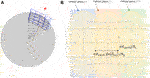FIGURE 4. Design of the satellite elliptical footprint. (A) Stereographic parametrization of the area covered by the satellite. (B) Illustration of the design process.

To design the sizes of the semiradiuses of the elliptical ROI, we will force the LEO constellation to cover the entire Earth. Thus, the equivalent flat problem we want to solve is to cover the entire Earth surface with ellipses centered in the vertices of a rectangular grid with spacing $πRearth/Ns$ in the first dimension and $πRearthsin(θop)/Np$ in the second dimension. Due to ellipses being convex and symmetric in both axes, this is equivalent to design ellipses containing the center points of the rectangular grid, as illustrated in Figure 4B. This condition can be written as

$(πRearth/NsRx)2+(πRearthsin(θop)/NpRy)2≤1.(12)$

An example of a semiradius pair that fulfills this condition is

$Rx=2πRearth/Ns, Ry=2πRearthsin(θop)/Np.(13)$

### 3.2 Beam Codebook Design

Our goal is to design a set of analog beampatterns or beam codebook, such that the set of beam footprints covers an ellipsoidal ROI as that described in the previous section. Depending on the location of the active users, some active beams will be selected from the set available in the codebook. We will consider a DFT-based analog precoder codebook structure, a popular solution when operating with planar arrays. In this case, the array response vectors in each dimension have a linearly increasing phase, like the columns in the DFT matrix. Therefore, the MIMO channel can be written in terms of array response vectors with this structure. DFT-based precoders match in this case the channel structure providing a good beamforming gain. Note that the column vectors in a DFT codebook generate a grid of beams (GoB) that can be used to span all angular directions, as illustrated in Figure 5 for a planar array with 16 panels (subarrays), and $7×5$ antenna elements per subarray.

FIGURE 5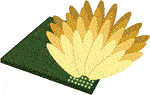FIGURE 5. Illustration of the GoB generated with a 2D DFT codebook with an oversampling factor of 2. Orthogonal beams are marked in red.

In particular, 5G New Radio defines what is called a Type II DFT codebook, where an oversampling factor is introduced in the definition of array steering vectors for azimuth and elevation (Miao et al., 2018; Ericsson, 2017a, b; Samsung, 2017; Technical Specification Group Radio Access Network, 2017a, b). The goal of the oversampling factor, which is fixed and equal to 4 in 5G NR, is to mitigate the loss of beamforming gain in directions between orthogonal beams (straddling loss). This way, beam orthogonality is lost in general, and only beams spaced the oversampling factor are orthogonal. Figure 5 shows the set of orthogonal beams marked in red when an oversampling factor equal to 2 has been used in each dimension.

In our design, we will also consider a 2D oversampled DFT codebook for each subarray, although the oversampling factor O is not fixed, allowing the adjustment of the number of beams that illuminate footprints of different sizes for some given RF resources. The elements of the block diagonal RF precoding matrix $FRF∈ℂNsat×Nb$ can be written in terms of this oversampled DFT codebook as

$[FRF]n,IRF(n)=e−i2λ([ksat]n,1θIRF(n),1+[ksat]n,2θIRF(n),2),(14)$

for ${(θm,1,θm,2)}m⊆{2π(n−1)ONxsub}nONxsub×{2π(n−1)ONysub}nONysub$. Note that the m-th element of the codebook is contained in the non-zero entries of the mth column of $FRF$, that is $[FRF]n,m=e−i2λ([ksat]n,1θm,1+[ksat]n,2θm,2)$. This expression is also proportional to the steering vector in the direction $v∈S2$ with $[v]1=θm,1π$ and $[v]2=θm,2π$. To create the codebook, we prune the complete oversampled DFT dictionary, keeping only those beam-patterns pointing to the elliptical ROI. The oversampling factor can be used to adapt the RF resources to the size of the ROI and is the key parameter that drives the tesselation of the coverage area. By increasing O, the beam footprint is reduced, and the number of beampatterns pointing to the desired ROI increases. The optimal value for O, in terms of provided SNR, allows the creation of as many beams pointing to the ROI as available RF chains. Note that when increasing the oversampling factor, the dictionary provides a higher SNR over the ROI because the straddling loss decreases, but the interference between adjacent beams increases, leading to a reduction of the SINR. Therefore, the oversampling factor has to be carefully selected to find the appropriate tradeoff between SNR and SINR. The inter-beam interference problem can be addressed by designing a suitable digital precoding matrix $FBB$ that enables full frequency reuse, or by allocating different carriers to users served by adjacent beams, as proposed in (Angeletti and De Gaudenzi, 2020).

Once the codebook is created, the cells are defined as the areas inside the elliptical ROI where a given beam provides a higher gain than any other beam in the available codebooks for all the subarrays. Note that the DFT nature of the designed codebook creates a rectangular tiling of the ROI. Section 5 includes examples of the beam footprints when this type of codebook is assumed on board.

## 4 Coverage and Spectral Efficiency Calculation

### 4.1 Coverage

When operating with a transmitting beam-pattern $f∈ℂNsat$ in the satellite beam codebook, and a receiving beam-pattern $w∈ℂNUT$, the RSS is given by

$RSS[W]=|wHHf|2=|γ|2||2||2.(15)$

Note that this expression depends on the user and satellite positions in $xu$ and $xsat$ respectively. The different terms in Eq. 15 can be identified with the terms in the link budget equation. Thus $GRX[dB]=10log10(||2)$, the transmitter gain $GTX[dB]=10log10(||2)$, and $10log10(|γ|2)$ comprises all the losses $LPcable[dB]+LPat[dB]+LPfs[dB]$.

To compute the combining gain at the UT side, we will consider the LoS direction to be known (in the system model we have assumed that the satellite position is known) and the Rician component of the channel to be unknown. Thus, the combiner $wu$ will be computed as the one maximizing $|〈au(v),w〉|2||w||2$ for the set of feasible $w$ as discussed in Section 2.1. The average gain associated to this combiner can be computed as $E(|〈au(v)+1KraR,wu〉|2||wu||2)$. Since $aR$ is Gaussian and independent of any other variable, we can write the average gain as

$E(|〈au(v)+1KraR,wu〉|2||wu||2)=E(|〈au(v),wu〉|2‖wu‖2)+1KrE(|〈aR,wu〉|2||wu||2).(16)$

Now, if we make use of the definition of $wu$ and consider the Rician component to be uniform, i.e. $Σ=I$, we obtain the final expression for the receive gain as

$E(maxw|〈au(v),w〉|2‖w2‖)+1Kr.(17)$

Since the LEO satellite is transmitting simultaneously through multiple beams with a gain $GnTX$, $n=1,…,Nb$, besides considering the RSS or the SNR, we also need to understand the impact of interference when computing the coverage. To this aim, we will compute the interference as the RSS of the signals transmitted through undesired beam-patterns

$I[dW]=PTX[dBW]−LPcable[dB]+GTXinterf[dB]−LPat[dB]−LPfs[dB]+GRX[dB](18)$

with $GnselectedTX$ the gain corresponding to the desired beampattern and $GTXinterf=∑n≠nselectedGnTX$. With these definitions, the signal to interference plus noise ratio (SINR) has the expression

$SINR=RSS[W]I[W]+σ2[W].(19)$

### 4.2 Throughput and Spectral Efficiency

We consider the throughput expression given by the capacity $BDLlog2(1+SINR)$ in bits per second. We also consider the throughput without interference $BDLlog2(1+SNR)$ as a bound to what can be achieved with inter-beam interference mitigation techniques. Assuming that frequency division duplexing mechanisms enable beam sharing among close users illuminated by the same beam, we will also consider the spectral efficiency with interference $log2(1+SINR)$ and without interference $log2(1+SNR)$ as performance metric.

## 5 Simulation Results

Unless otherwise specified, we consider a LEO satellite orbiting at a 1,300 km height, covering an ellipsoidal ROI with semiradius 534.1 and 170.5 km. This area has been designed following the procedure described in 3.1 for a LEO constellation with 83 orbits with an inclination of $53∘$ and 53 satellites in each orbit. The downlink operates in the Ku band with a carrier frequency $fDL=11.45GHz$ and a bandwidth $BDL=250MHz$. A single polarization is assumed in the simulations.

To understand the role of the oversampling factor O in the beam codebook presented in Section 3.2, we show in Figure 6 different mesh designs covering the elliptical ROI for a $128×64$ satellite phased array and 8 RF chains in each dimension. Figure 6A shows the results when $O=2.49$, while Figure 6B shows the obtained rectangular mesh when $O=2$. Note that with $O=2$, only 39 out of 64 potential beams are used to illuminate the ROI, resulting in a very inefficient usage of the RF resources.

FIGURE 6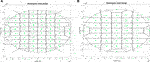FIGURE 6. Examples of rectangular mesh covering an elliptical ROI when using the oversampled DFT beam codebook: (A) with $O=2.49$; (B) with $O=2$. Please note that this ROI is a toy example and does not correspond to the designed one for the simulations.

For the next simulations we will assume that the satellite phased arrays consist of $5×3$ sub-arrays of size $12×24$, with a total of $60×72$ antennas. Note that while we are arranging the sub-arrays into a larger plannar antenna array structure, each subarray will be transmitting an independent signal. We found out that, for this number of antennas and RF-chains, with an oversampling factor of 1.2 we can adjust all the available beams to the size of the ROI. The remaining system parameters are summarized in Table 1.

TABLE 1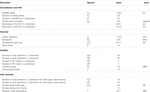TABLE 1. System parameters for the simulation of the downlink of a LEO SatCom system operating in the Ku band.

To understand the impact of different antennas at the user terminal, we will consider both the UPA and the leaky wave antenna (denoted as CRLH-LW) to have a number of elements equal to $24×24$. Beamforming with the planar antenna array at the user side introduces a gain of 27.6 dB. The gain provided by the leaky wave antenna is a function of the leakage factor, as illustrated in Figure 7A. The dependance of the antenna developed by Kymeta with the elevation angle (Kymeta, 2019) is also shown in Figure 7A.

FIGURE 7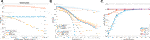FIGURE 7. Gain provided by the different antenna terminals: (A) Gain with perfect beam alignment. (B) Gain under misalignment. (C) Gain under phase quantization.

We consider now the effect of beam misalignment on the gain through Monte Carlo simulation for the phased antenna array and the leaky wave antenna. Figure 7B shows that, on average, the gains stay within reasonable levels, but the minimum values become critical under an error greater than $4deg$. The effects of phase shifter quantization for the phased array in a hybrid architecture using the beamforming method proposed in (Palacios et al., 2016) are shown in Figure 7C. It can be seen that a hybrid architecture offers a much better performance than an analog one under phase quantization, but both perform very similarly when the phase shifters have a high resolution.

Next, we analyze the coverage of the elliptical ROI provided by the beam codebook at the satellite when the UPA is considered as antenna terminal. As shown in Section 4.1, $LPfs$, $GTX$ and $GTXinterf$ depend on the user location. The coverage provided by the satellite’s codebook in terms of SNR and SINR can be seen in Figure 8. While the SNR shows a good coverage of the ROI for an oversampling factor of 1.2, when visualizing the SINR we can observe areas with high interference. This is a consequence of using the DFT-type codebook employed in 5G NR, since its associated rectangular mesh creates points that are covered with the same gain by 4 different satellite beams. When selecting one of the beams, the other three will create a strong interference, leading to a SINR not larger than $−4.77[dB]$. Furthermore, the design of static codebooks will always lead to points in which at least 3 beam-patterns have equal gain, thus leading to a SINR never larger than $−3[dB]$.

FIGURE 8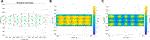FIGURE 8. (A) Cells associated to the different beampatterns; point marked in green show the location where the beampattern gain is maximum. (B) SNR when using the best beam for each cell. (C) SINR when using the best beam for each cell.

This SNR and SINR translate into the spectral efficiency shown in Figure 9. Note that there is a significant inter-beam interference when operating with DFT-type codebooks as proposed in LTE and 5G NR. Future work needs to be developed to design alternative analog codebooks and hybrid beamforming strategies at the satellite side to reduce inter-beam interference.

FIGURE 9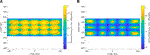FIGURE 9. (A) Spectral efficiency when ignoring interference. (B) Spectral efficiency when considering interference.

To further extend the coverage analysis, in Figure 10 we see how the SNR, SINR and their corresponding spectral efficiencies are distributed over the ROI as CDF curves. It is easy to appreciate the huge impact of inter-beam interference, hinting how important the design of good interference mitigation techniques is for satellite communications.

FIGURE 10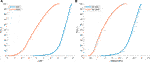FIGURE 10. Cumulative distribution function inside the ROI of: (A) SNR and SINR. (B) Spectral efficiency computed for the SNR and SINR.

Figure 11 shows the satellite’s angular speed relative to the user and the Doppler effect due to the satellite’s movement as a function of the user’s relative location. The relative angular speed shows significantly small values. This means that the beampattern switching requirements of a given antenna design that enable tracking of the satellite’s position for beamforming are not particularly challenging. Note that this holds when tracking the satellite direction, not its corresponding azimuth and elevation angles. If the angular relative speed is translated into a maximum azimuth and elevation angular variation with time, the azimuth angle can present sharp changes, as illustrated in Section. 2.2.2.

FIGURE 11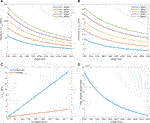FIGURE 11. (A–C) Doppler effect for the central frequency caused by the satellite’s movement, Earth rotation and UT movement respectively. (D) Satellite’s angular relative speed with respect to the user terminal.

Finally, we analyze the SNR degradation over time due to the satellite’s movement for a user initially located at the center of the elliptical ROI. We have considered a beam selection strategy based on maximizing SNR. Figure 12A shows an example of different satellite positions and associated selected beams. Figure 12B shows the SNR corresponding to the different satellite positions illustrated in (a). It can be concluded that, for this codebook design and system parameters, the user is illuminated by the same beam for a period of around 30 s. After that, another beam has to be selected to keep a proper gain. Note that even after updating the best beam, there is a non-negligible gain loss due to the satellite movement, as also illustrated in Figure 12B.

FIGURE 12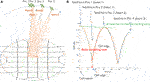FIGURE 12. (A) Example of different satellite positions and associated beams. (B) SNR degradation over time due to the satellite’s movement.

## 6 Conclusion

Research on integrating LEO-based satellite networks with terrestrial networks is still in its infancy. We implemented a complete simulator of the downlink of a massive MIMO LEO SatCom system to understand the physical layer challenges and evaluate a hybrid beamforming strategy based on an analog codebook. We designed the satellite footprint as an elliptical ROI given the number of orbital planes and number of satellites per orbit. We also designed a static DFT-type codebook, as commonly used in current cellular standards, to adjust the beamwidth and RF resources to the size of the satellite footprint by means of an interpolation factor. Simulation results show the relevance of the antenna terminal design to achieve an acceptable SNR. Our numerical experiments also show that DFT codebooks introduce a high inter-beam interference which reduces the ability of the system to provide a high spectral efficiency at all locations inside the ROI. Designing fully or partially connected hybrid beamforming architectures with per antenna power constraints and a digital precoder that mitigates inter-beam interference is an open research challenge that needs to be addressed to overcome the SINR performance of current approaches. The design of low complexity dynamic codebooks that better adapt the resources to the specific location of the users and reduce the SNR loss due to the satellite movement is a critical challenge. Our simulator also shows that the variation of the relative angular speed between the satellite and the UT is smooth when working with spherical coordinates. This leads to the need of designing beam tracking methods for the UT that track the satellite position rather than its variation in elevation and azimuth. Our numerical experiments also show the effectiveness of exploiting satellite position information at the UT side to avoid the need of channel estimation and tracking, which will provide old estimates due to the long transmission delay. Finally, an accurate model for the error in the satellite’s position and trajectory is also necessary to better understand its impact in the performance of the satellite beam tracking strategies.

## Data Availability Statement

The original contributions presented in the study are included in the article/supplementary material, further inquiries can be directed to the corresponding author.

## Author Contributions

JP and NG-P contributed to the design and development of the investigation, including mathematical derivations and code for the simulations. NG-P and CM provided technical direction. JP and NG-P wrote the draft of the manuscript. CM, TS and C-HW participated in technical discussions of the results and provided comments of the manuscript.

## Funding

This work has been partially funded by Toyota Motor North America.

## Conflict of Interest

Authors TS and C-HW were employed by the company Toyota Motor North America.

The remaining authors declare that the research was conducted in the absence of any commercial or financial relationships that could be construed as a potential conflict of interest.

## Publisher’s Note

All claims expressed in this article are solely those of the authors and do not necessarily represent those of their affiliated organizations, or those of the publisher, the editors and the reviewers. Any product that may be evaluated in this article, or claim that may be made by its manufacturer, is not guaranteed or endorsed by the publisher.

## References

Angeletti, P., and De Gaudenzi, R. (2020). A Pragmatic Approach to Massive MIMO for Broadband Communication Satellites. IEEE Access 8, 132212–132236. doi:10.1109/access.2020.3009850

De Gaudenzi, R., Angeletti, P., Petrolati, D., and Re, E. (2020). Future Technologies for Very High Throughput Satellite Systems. Int. J. Satell Commun. Netw. 38, 141–161. doi:10.1002/sat.1327

del Portillo, I., Cameron, B. G., and Crawley, E. F. (2019). A Technical Comparison of Three Low Earth Orbit Satellite Constellation Systems to Provide Global Broadband. Acta Astronautica 159, 123–135. doi:10.1016/j.actaastro.2019.03.040

Devillers, B., and Pérez-Neira, A. (2011). “Advanced Interference Mitigation Techniques for the Forward Link of Multi-Beam Broadband Satellite Systems,” in 2011 Conference Record of the Forty Fifth Asilomar Conference on Signals, Systems and Computers (ASILOMAR), 1810–1814. doi:10.1109/ACSSC.2011.6190334

[Dataset] Ericsson, (2017a). On Basis Design for Type I and Type II Codebooks.

Ericsson, (2017b). Type II CSI for Beamformed CSI-RS and Hybrid Operation. Tech. Rep. R1-1708699. Hangzhou, China. Meeting RAN1#89, 3GPP.

Kim, J., Yun, M. Y., You, D., and Lee, M. (2020). “Beam Management for 5G Satellite Systems Based on NR,” in 2020 International Conference on Information Networking (ICOIN), 32–34. doi:10.1109/icoin48656.2020.9016571

Kodheli, O., Guidotti, A., and Vanelli-Coralli, A. (2017). “Integration of Satellites in 5G through LEO Constellations,” in GLOBECOM 2017 - 2017 IEEE Global Communications Conference, 1–6. doi:10.1109/glocom.2017.8255103

[Dataset] Kymeta (2019). Link Budget Calculations for A Satellite Link with an Electronically Steerable Antenna Terminal.

Mehdipour, A., and Eleftheriades, G. V. (2014). Leaky-Wave Antennas Using Negative-Refractive-Index Transmission-Line Metamaterial Supercells. IEEE Trans. Antennas Propagat. 62, 3929–3942. doi:10.1109/TAP.2014.2322882

Mehdipour, A., Sazegar, M., and Stevenson, R. (2019). “Broadband WAIM Metasurface Structure for Electronically Beam Scanning Holographic Antenna for Ku-Band Satellite Communications,” in 2019 IEEE International Symposium on Antennas and Propagation and USNC-URSI Radio Science Meeting, 429–430. doi:10.1109/APUSNCURSINRSM.2019.8888376

Miao, H., Mueck, M. D., and Faerber, M. (2018). “Amplitude Quantization for Type-2 Codebook Based CSI Feedback in New Radio System,” in 2018 European Conference on Networks and Communications (EuCNC). 1–9. doi:10.1109/EuCNC.2018.8442609

Palacios, J., De Donno, D., Giustiniano, D., and Widmer, J. (2016). “Speeding up mmWave Beam Training through Low-Complexity Hybrid Transceivers,” in 2016 IEEE 27th Annual International Symposium on Personal, Indoor, and Mobile Radio Communications (PIMRC), 1–7. doi:10.1109/PIMRC.2016.7794709

Samsung (2017). WF on Type I and II CSI Codebooks. Tech. Rep. R1-1709232. Hangzhou, China. Meeting RAN1#89, 3GPP.

Sikri, D., and Jayasuriya, R. M. (2019). Multi-Beam Phased Array with Full Digital Beamforming for SATCOM and 5G. Microwave J. 60 (4), 64–79.

[Dataset] Technical Specification Group Radio Access Network (2017a). TR38.211. NR; Physical Channels and Modulation (Release 15).

[Dataset] Technical Specification Group Radio Access Network (2017b). TR38.214. NRPhysical Layer Procedure for Data (Release 15).

[Dataset] Technical Specification Group Radio Access Network (2019). TR38.821. Solutions for NR to Support Non-terrestrial Networks (NTN) (Release 16).

Xia, S., Jiang, Q., Zou, C., and Li, G. (2019). Beam Coverage Comparison of LEO Satellite Systems Based on User Diversification. IEEE Access 7, 181656–181667. doi:10.1109/access.2019.2959824

You, L., Li, K.-X., Wang, J., Gao, X., Xia, X.-G., and Ottersten, B. (2020). Massive MIMO Transmission for LEO Satellite Communications. IEEE J. Select. Areas Commun. 38, 1851–1865. doi:10.1109/jsac.2020.3000803

Keywords: beam codebook, massive MIMO, LEO satellite communications, non-terrestrial networks, hybrid beamforming

Citation: Palacios J, González-Prelcic N, Mosquera C, Shimizu T and Wang C-H (2021) A Hybrid Beamforming Design for Massive MIMO LEO Satellite Communications. Front. Space Technol. 2:696464. doi: 10.3389/frspt.2021.696464

Received: 16 April 2021; Accepted: 08 July 2021;
Published: 27 September 2021.

Edited by:

Tomaso De Cola, Helmholtz Association of German Research Centers (HZ), Germany

Reviewed by:

Jue Wang, Nantong University, China
Nicolò Mazzali, European Space Research and Technology Centre (ESTEC), Netherlands

Copyright © 2021 Palacios, González-Prelcic, Mosquera, Shimizu and Wang. This is an open-access article distributed under the terms of the Creative Commons Attribution License (CC BY). The use, distribution or reproduction in other forums is permitted, provided the original author(s) and the copyright owner(s) are credited and that the original publication in this journal is cited, in accordance with accepted academic practice. No use, distribution or reproduction is permitted which does not comply with these terms.

*Correspondence: Nuria González-Prelcic, ngprelcic@ncsu.edu